edHelper subscribers - Create a new printable

Number of Keys
 Select the number of different printables: 1 key 2 keys 3 keys 4 keys 5 keys

Number of Pages (for each key)
 Select the number of pages: 1 page 2 pages 3 pages 4 pages

Include an answer key (answer keys will be at the end of the printable)

Not a subscriber?  Sign up now for the subscriber materials!
Sample edHelper.com - Math Review Worksheet

 Name _____________________________Date ___________________
Mixed Review
Find the measure of the angle.
 1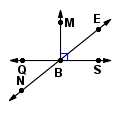m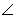NBQ = _________mNBS = 1412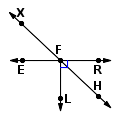mHFR = _________mXFE = 433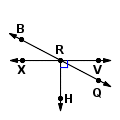mQRH = _________mQRV = 28Add or subtract. Write the answer in simplest form.
4.
3  +
 14

5.
 612
+  15

6.
18  +
 313

Circle the equivalent fraction.
7.
 13

 26
 2048
 31
 111
8.
 27

 28
 828
 611
 116

 Key #2
Simplify.
 9 7 +  (4 + 1)
 10 8 - 3 - 2 + 3
 11 (9 - 4)  - 5 +  (2 + 4)
 12 6 -  (3 + 1)

Use the clue to fill in the missing digit.
 13 The number 76is divisible by 19.
 14 The number63 is divisible by 9.
 15 The number 22 is divisible by 17.
 16 The number 320is divisible by 6.
 17 The number 538is divisible by 18.
 18 The number 48 is divisible by 4.
 19 The number 263is divisible by 3.
 20 The number 919is divisible by 10.

Draw and label an example of each.
21.JA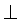BG
22.CF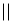TP
23.
 point R

Write each fraction or mixed number as a decimal.  Use a bar to show a repeating decimal.
24.
 427
25.
 170
26.
 -3 1532
27.
 396
28.
 1 2363
29.
 1966
30.
 6 1365
31.
 3648

edHelper subscribers - Create a new printable

Number of Keys
 Select the number of different printables: 1 key 2 keys 3 keys 4 keys 5 keys

Number of Pages (for each key)
 Select the number of pages: 1 page 2 pages 3 pages 4 pages